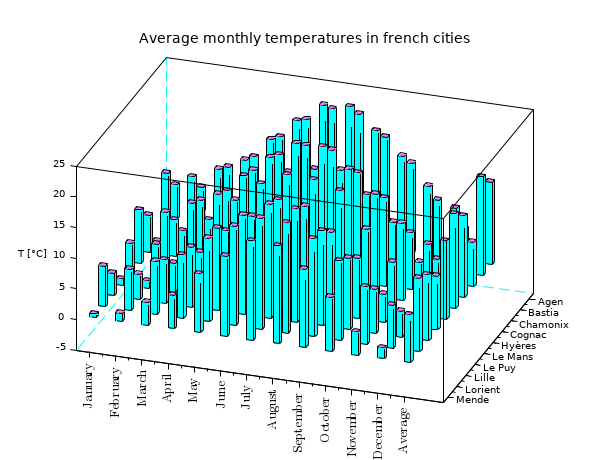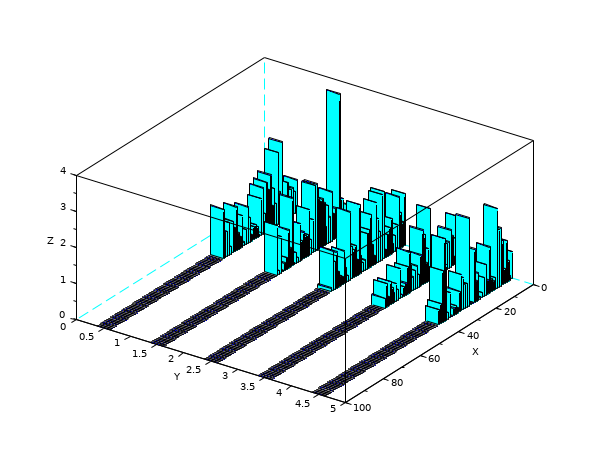Scilab Home page | Wiki | Bug tracker | Forge | Mailing list archives | ATOMS | File exchange
Change language to: Français - Português - 日本語 - Русский
Scilab Help >> Graphics > 3d_plot > hist3d

# hist3d

3D representation of a histogram (obsolete)

### Syntax

```hist3d(mtx,[theta,alpha,leg,flag,ebox])
hist3d(mtx,<opt_args>)
hist3d(list(mtx,x,y),[theta,alpha,leg,flag,ebox])
hist3d(list(mtx,x,y),<opt_args>)```

### Arguments

mtx

matrix of size `(m,n)` defining the histogram `mtx(i,j)=F(x(i),y(j))`, where `x` and `y` are taken as `0:m` and `0:n`.

list(mtx,x,y)

where `mtx` is a matrix of size `(m,n)` defining the histogram `mtx(i,j)=F(x(i),y(j))`, with `x` and `y` vectors of size `(1,m+1)` and `(1,n+1)`.

<opt_args>

This represents a sequence of statements `key1=value1`, `key2=value2`,... where `key1`, `key2,...` can be one of the following: `theta`, `alpha`, `leg`, `flag`, `ebox`. See plot3d.

theta,alpha,leg,flag,ebox

see plot3d.

### DescriptionUp to Scilab 6.0, `hist3d()` features are actually some `bar3d()` ones. In Scilab 6.1.0, `bar3d()` is introduced as a clone of `hist3d()`. From now on, please use `bar3d()` in replacement of the former `hist3d()`. `hist3d()` will be redefined in Scilab 6.2.0 to actually support 3D histogram features.

`hist3d` represents a 2d histogram as a 3D plot. The values are associated to the intervals ```[x(i) x(i+1)[ X [y(i) y(i+1)[``` .

Enter the command `hist3d()` to see a demo.

### Examples

`hist3d();``hist3d(10*rand(10,10));````Z = zeros(100,5);
A = abs(rand(40,5));
Z(1:40,:) = A;
scf();
hist3d(Z);
isoview off``````Z = zeros(100,5);
A = abs(rand(40,5));
Z(1:40,:) = A;
Index = find(Z==0);
Z(Index) = %nan;
scf();
hist3d(Z);
isoview off``````Z = zeros(100,5);
A = abs(rand(40,5));
Z(1:40,:) = A;
A = abs(rand(10,5));
Z(91:100,:) = A;
scf();
hist3d(Z);
isoview off```### History

 Version Description 6.1.0 The current implementation of hist3d() is declared obsolete. Use bar3d() instead.